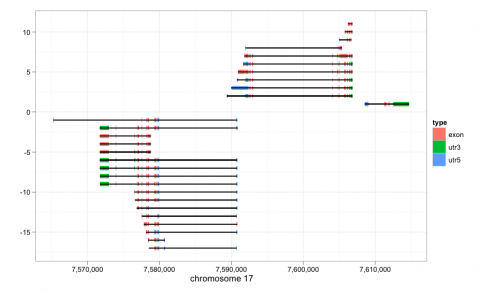[This article was first published on rforcancer, and kindly contributed to R-bloggers]. (You can report issue about the content on this page here)
Want to share your content on R-bloggers? click here if you have a blog, or here if you don't.

I’m writing a new package that will create nice publication quality graphics of genome information. It’s really an adaptor sitting between the biomaRt and ggplot2 packages. Here is the code so far:

```## this function integrates 3 steps to creating a genome plot
## 1 query bioMart to get a GFF like data-frame from ensembl
## 2 add new elements to the gff to make it plot with ggplot
## 3 plot it with ggplot2
## the reason to split it like this is so that basic users may use this simple function
## and advanced users can try custom queries or altered plotting themes

gnmplot= function(filters='hgnc_symbol', values=c('TP53', 'WRAP53', 'EFNB3'), species='human')
{

gff= getGFF(filters=filters, values=values, species=species)

gff.new=alterGFF(gff)

gnm.plot=gffplot(gff.new)

return(gnm.plot)

}

getGFF=function(filters=filters, values=values, species=species)
{

## getGFF uses biomaRt getBM() to query ensembl and return a GFF like dataframe
## to integrate with alterGFF() the attributes returned must be stable
## however with a reasonable understanding of biomaRt you can use different filters and values

require(biomaRt)

## built-in shortcuts for species names
if(species=='human') species.str="hsapiens_gene_ensembl"
if(species=='mouse') species.str="mmusculus_gene_ensembl"
if(species=='drosophila') species.str="dmelanogaster_gene_ensembl"
if(!any(species==c('human', 'mouse', 'drosphila'))) species.str=species

## we are restricted to using ensembl datbases
mart<-useDataset(species.str, useMart("ensembl"))

gff=getBM(attributes=c('chromosome_name','ensembl_gene_id', 'ensembl_transcript_id','5_utr_start', '5_utr_end',
'3_utr_start', '3_utr_end', 'start_position','end_position', 'exon_chrom_start', 'exon_chrom_end', 'strand'),
filters = filters, values = values, mart=mart)

return(gff)
}

alterGFF= function(gff=gff)
{
## this function adds columns to a getGFF() dataframe fro plotting by ggplot2
## this function requires specific named columns so is very customisable

## R does not like variables or column names that begin with numbers e.g. 5_utr_start

colnames(gff)[4:7]=c('utr5_start', 'utr5_end', 'utr3_start', 'utr3_end')

## categorise which rows are exons and which utrs and add a column
type=rep('exon', dim(gff))
type[which(!is.na(gff\$utr5_start))]='utr5'
type[which(!is.na(gff\$utr3_start))]='utr3'
gff=data.frame(gff,type=type)

## to plot a line the length of each gene I need to add this data to each row
## so I need to find the max and min exons for each transcript
## the merge them back into each row of the original data
ag.min=aggregate(gff, list(gff\$ensembl_transcript_id), function(x)min(as.numeric(x),na.rm=T))
ag.max=aggregate(gff, list(gff\$ensembl_transcript_id), function(x)max(as.numeric(x),na.rm=T))
ag=data.frame(ensembl_transcript_id= ag.min\$Group.1, start=ag.min\$exon_chrom_start, end=ag.max\$exon_chrom_end, strand=ag.max\$strand)
ag= ag[order(ag\$start),]

## We are adding a y offset for each transcript element
## So we have to see whether they are overlapping i.e. if so it needs a new row	##
## If it is on the pos or neg strand											##
## good luck to anyone who wants to try unlooping this							##

yneg=-1; ypos=1; mem=vector(); y=vector()
for(i in 1:length(ag\$start))
{
genevec=ag\$start[i]:ag\$end[i]
ynew=ifelse(length(intersect(genevec, mem))>0, TRUE, FALSE)
strandpos=ifelse(ag\$strand[i]>0, TRUE, FALSE)

if(ynew && strandpos)
ypos=ypos+1
if(ynew && !strandpos)
yneg=yneg-1
if(!ynew && strandpos)
ypos= 1
if(!ynew && !strandpos)
yneg= -1

if(strandpos)
y[i]=ypos
if(!strandpos)
y[i]=yneg

mem=c(mem,genevec)
}

ag=data.frame(ag,y=y)

## here we are merging the start end and the y offset for each transcript back into the original data
## so it is associated with every feature-row
gff=merge(ag,gff)

## to plot the line we also need to show arrow direction which requires an option first or last
ardir=ifelse(gff\$strand==1,'last','first')
gff=data.frame(gff,ardir=ardir)

## OK there is an annoying glitch- that if you use strand for facet_grid then -1 will be on top
## the easiest workround is to create a dummy char variable that is alphabetically the right way!!!!!

strandChar=gff\$strand
strandChar[strandChar==1]<-'forward'
strandChar[strandChar==(-1)]<-'reverse'
gff.new=data.frame(gff, strandChar=strandChar)

return(gff.new)
}

gffplot=function(gff.new=gff.new)
{
## the ggplot package is used to create genomeplots
## geom_rect is used to create each seq element (e.g. UTRs, miRNAs, exons) i.e. with a enesembl_transcript ID
## geom_segment is used to create a line that links from the start to end of each gene or miRNA i.e. with a ensembl_gene_id
##I buid the plot in stages as the options are a bit lengthy

require(ggplot2)

gnm1=ggplot(aes(xmin=exon_chrom_start, xmax=exon_chrom_end, ymin=y+0.25, ymax= y-0.25, fill=type, label=ensembl_transcript_id), data=gff.new)

gnm2= gnm1+ geom_rect()+geom_segment(aes(x=start,xend=end, y=y, yend= y))+theme_bw()+facet_grid(strandChar~., scales='free_y')

gnm.plot= gnm2+ ylab('')+xlab(paste('chromosome',gff\$chromosome_name))+scale_x_continuous(formatter = "comma")

return(gnm.plot)

```

and here is an example plot: